In this chapter, you will learn how to define your own constraints propagation mechanism and how to make them efficient and incremental.

## Constraint’s semantic¶

We will start by specifying the semantic of the constraint. It is defined by:

• A constant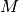.

• Two variables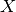and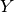withthe result of the operation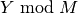.

let’s see how to implement this constraint it the Artelys Kalis library framework:

## Implementation of the constraint¶

The `KUserConstraint` is the base class of all the user defined constraints in Artelys Kalis. When you want to define your own constraint, you have to create a new class that will inherit from the `KUserConstraint` class. The `KUserConstraint` class contains six kind of interfaces:

```class KUserConstraint: public KConstraint
{
public:
KIntVarArray _vars;

/// Constructor for unary constraints
KUserConstraint(KIntVar &v1);

/// Constructor for binary constraints
KUserConstraint(KIntVar &v1,KIntVar &v2);

/// Constructor for n-ary constraints
KUserConstraint(KIntVarArray &vars);

/// Copy constructor
KUserConstraint(const KUserConstraint & toCopy);

// destructor
virtual ~KUserConstraint();

/// virtual method called when the domain of some or several variables has changed
virtual void propagate(void) = 0;

/// virtual method called upon initialization of the constraint
virtual void awake(void) = 0;

/// virtual method called when the lower bound of var has been raised
virtual void awakeOnInf(KIntVar & var);

/** virtual method called when the upper bound of var has been lowered
@param var the variable with modified domain
*/
virtual void awakeOnSup(KIntVar & var);

/** virtual method called when the variable var has been instantiated
@param var the variable with modified domain
*/
virtual void awakeOnInst(KIntVar & var);

/** virtual method called when the value removedValue has been removed from the domain of var
@param var the variable with modified domain
@param removedValue the value that has been removed from the domain of var
*/
virtual void awakeOnRem(KIntVar & var,int removedValue);

/** virtual method called when the domain of variable var has changed
@param var the variable with modified domain
*/
virtual void awakeOnVar(KIntVar & var);

/** virtual method for use within boolean connectors
@return CTRUE whenever the constraint is definitively satisfied
@return CFALSE whenever the constraint is definitively violated
@return CUNKNOWN otherwhise
*/

virtual void print(void)=0;
};
```
• Several constructors that need to be called by the subclasses constructors;

• An awake and a propagate method that need to be implemented;

• Severals `awake()` methods that may be implemented for an incremental propagation scheme (`awakeOnInf()`, `awakeOnSup()`, `awakeOnInst()`, `awakeOnRem()`, `awakOnVar()`)

• A `print()` method that needs to be implemented;

• An `askIfEntailed()` method;

• A `getCopy(KProblem*)` method.

The purpose of these methods will be explained later. Let’s first start by creating a class named `ModuloConstraintXYM` that inherit from the `KUserConstraint` interface. We will implement one constructor, the awake and propagate methods, the `awakeOnInst()` method, the print and the `askIfEntailed()` methods:

```//*********************************************************************************
// Definition of the constraint X == Y mod M.
//*********************************************************************************
class ModuloConstraintXYM: public KUserConstraint
{
private:
int _M;
KIntVar * _VX;
KIntVar * _VY;

public:
ModuloConstraintXYM(KIntVar &X, KIntVar &Y, const int modulo);
virtual void awake(void);
virtual void propagate(void);
virtual void awakeOnInst(KIntVar & var);
virtual void print();
virtual KConstraint* getCopyPtr(const KProblem& problem) const;
};
```

## Construction of the constraint¶

To create the constraint, we need to define a constructor for the class that will initialize the variables and the data linked to the constraint. Note that we need to explicitly make a call to the constructor for binary constraints of the super-class `KUserConstraint`.

```ModuloConstraintXYC::ModuloConstraintXYC(KIntVar &X, KIntVar &Y, const int modulo): KUserConstraint(X,Y)
{
// note that you must call the superclass constructor
_M = modulo;
_VX = &X;
_VY = &Y;
}
```

Now that we have implemented a constructor for the constraint, we will look in detail the purpose and the implementation of the awake and propagate methods.

## Constraint’s events¶

Two kind of events can be thrown to a constraint: events linked to the constraint itself and events linked to a specific variable of the constraint. We will describe here the first kind of event.

Events linked to the constraint can be of two kinds:

• Awake events which purpose is to initialize the constraint and do an initial propagation.

• Propagate events that occur when some unknown changes have been applied to the domain of the variables involved in the constraint.

### The awake method¶

Upon initialization of the constraint, it is possible to deduce that thevariable representing the result of the operation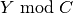is bounded by 0 and M-1:

```void ModuloConstraintXYC::awake()
{
// Trivial deduction: 0 <= Y mod M < M
_VX->setInf(0);
_VX->setSup(_M-1);

// Now do some AC3 like propagation (arc consistency)
propagate();
}
```

Note that this method can throw a contradiction since it updates the domains of the variables that may be empty after the deductions.

### propagate method¶

Now we have to implement the propagate method that will be triggered by any modifications of the domains of the variables (This execution can be forced by calling the constAwake method of `KUserConstraint`). In order to find impossible values we will use an AC3 like algorithm that try to find a supporting value (in the domain of the other variable) for each values in the domain of one variable. For example ifcan take value 1,2 and 3 andcan take value in 1,2,4 and theconstant equal 4, the value ‘3’ ofhas no supporting value in the domain ofsince neither 1 mod 4, 2 mod 4 and 4 mod 4 equal 3. Reciprocally, the value 4 in the domain ofhas no supporting value in the domain ofsince 0 do not belong to the domain of. Hence we can deduce that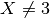and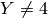. The following algorithm implement an AC3 like propagation for the constraint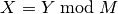:

```void ModuloConstraintXYC::propagate()
{
int vx, vy;

// Now do some AC3 like propagation
// Propagating from X to Y
for ( vy = _VY->getInf();vy<=_VY->getSup();vy ++)
{
bool support = false;
for ( vx = _VX->getInf();vx<=_VX->getSup();vx ++)
{
if ( vx == vy % _M)
{
support = true;
break;
}
}

if ( !support)
{
_VY->remVal(vy);
}
}

// Propagating from Y to X
for ( vx = _VX->getInf();vx<=_VX->getSup();vx ++)
{
bool support = false;
for ( vy = _VY->getInf();vy<=_VY->getSup();vy ++)
{
if ( vx == vy % _M)
{
support = true;
break;
}
}

if ( !support)
{
_VX->remVal(vx);
}
}
}
```

## Events on variables¶

The second kind of events that can be triggered in a constraint is events linked to the variables of the constraint (following decisions taken by the search algorithm or by the filtering of other constraints of the problem). The differents methods related to these events are:

• `awakeOnInf` that is triggered upon raising of the lower bound of one variable;

• `awakeOnSup` that is triggered upon lowering of the upper bound of one variable;

• `awakeOnRem` that is triggered upon deletion of a value from the domain of one variable;

• `awakeOnInst` that is triggered upon instantiation of one variable;

• `awakeOnVar` that is triggered upon unknown modification of the domain of one variable.

When these methods are not overloaded, the default behavior of the `KUserConstraint` is to call the propagate method. Overloading one of the `awakeOnXXX()` methods allows the user to implement a more incremental propagation scheme by giving some information about what has changed since the last call. Note that you can also use the `constAwake()` method inside one of the `awakeOnXXX()` methods (in the `propagate()` method, the call would cause an infinite loop) to force the constraint engine to call the propagate method of this constraint later (Usually when you want to delay the propagation or do dot want to react incrementally to one specific event). For our constraint we will only overload the `awakeOnInst()` method. (The other events will trigger the `propagate()` method).

### awakeOnInst method¶

This method is called when a specific variable is instantiated to a specific value. The variable is passed in parameter of the method. The instantiation value can be found by calling the `getValue()` method of the variable. Since one of the variable is instantiated, the loop on the values of the domain of this variable is useless. Hence we can define a lighter propagation algorithm looking only for one supporting value for the instantiation value of the instantiated variable thus avoiding the loop on the values and the filtering on the other variable:

```void ModuloConstraintXYC::awakeOnInst(KIntVar & var)
{
int v;

if ( var.isEqualTo(*_VX) )
{
// X has been instantiated , removing all values in domain of Y inconsistent with the constraint
for ( v = _VY->getInf();v<=_VY->getSup();v ++)
{
if (_VX->getValue() != v % _M)
{
_VY->remVal(v);
}
}
}
else if ( var.isEqualTo(*_VY) )
{
// Y has been instantiated , removing all values in domain of X inconsistent with the constraint
for ( v = _VX->getInf();v<=_VX->getSup();v ++)
{
if ( v != _VY->getValue() % _M)
{
_VX->remVal(v);
}
}
}
}
```

The `print()` method is used by the constraint engine to print the name of the constraint to the standard output. It is mainly used by the `KProblem::print()` method to print the problem.

```void ModuloConstraintXYC::print()
{
printf("%s == %s mod %i",_VX->getName(),_VY->getName(),_M);
}
```

The `askIfentailed()` method is used by composite constraints such as `KImplies`, `KGuard`, `KEquiv`, etc. The return value of the method correspond to one of the three following values depending on the status of the constraint:

• `KUserConstraint::CTRUE` when the constraint is definitively satisfied

• `KUserConstraint::CFALSE` when the constraint is definitively violated

• `KUserConstraint::CUNKNOWN` when the status of the constraint is unknown

This listing show an implementation of this method for the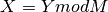constraint :

```int ModuloConstraintXYC::askIfEntailed()
{
if (_VX->getIsInstantiated() && _VY->getIsInstantiated() )
{
// Here X and Y have been instantiated so we now if the constraint is satisfied or violated
if ( _VX->getValue() == _VY->getValue() % _M )
{
// the constraint hold since X == Y mod M
return CTRUE;
}
else
{
// the constraint does not hold since X != Y mod M
return CFALSE;
}
}
else
{
// X or Y is not yet instantiated so we do not know yet if the constraint holds
return CUNKNOWN;
}
}
```

The `getCopyPtr(KProblem*)` method is used by Artelys Kalis to obtain an instance copy of the constraint (see Section 9.4) for details about implementation of this method).

```KConstraint* ModuloConstraintXYC::getCopyPtr(const KProblem& problem) const
{
return new ModuloConstraintXYC(*problem.getCopyPtr(_VX), *problem.getCopyPtr(_VY), _M);
}
```